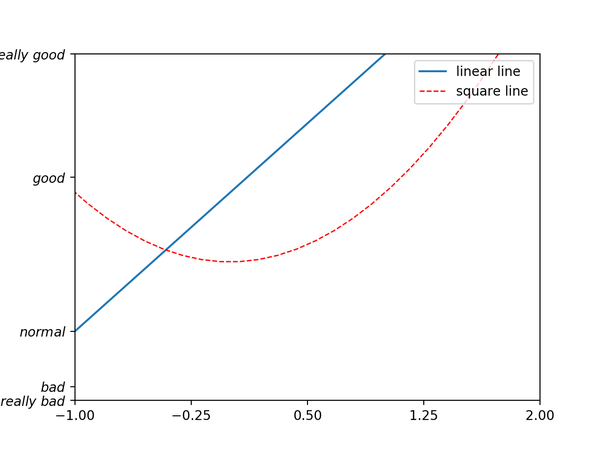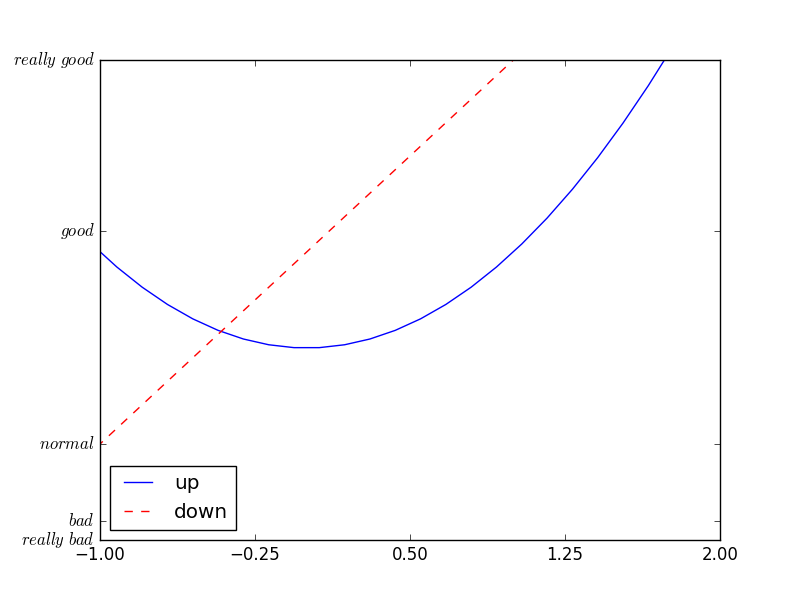# Matplotlib Legend 图例

882℃

## 添加图例

matplotlib 中的 `legend` 图例就是为了帮我们展示出每个数据对应的图像名称. 更好的让读者认识到你的数据结构.

``````import matplotlib.pyplot as plt
import numpy as np

x = np.linspace(-3, 3, 50)
y1 = 2*x + 1
y2 = x**2

plt.figure()
#set x limits
plt.xlim((-1, 2))
plt.ylim((-2, 3))

# set new sticks
new_sticks = np.linspace(-1, 2, 5)
plt.xticks(new_sticks)
# set tick labels
plt.yticks([-2, -1.8, -1, 1.22, 3],

``````# set line syles
l1, = plt.plot(x, y1, label='linear line')
l2, = plt.plot(x, y2, color='red', linewidth=1.0, linestyle='--', label='square line')``````

`legend`将要显示的信息来自于上面代码中的 `label`. 所以我们只需要简单写下一下代码, plt 就能自动的为我们添加图例.

``plt.legend(loc='upper right')``## 调整位置和名称

``plt.legend(handles=[l1, l2], labels=['up', 'down'],  loc='best')```````` 'best' : 0,
'upper right'  : 1,
'upper left'   : 2,
'lower left'   : 3,
'lower right'  : 4,
'right'        : 5,
'center left'  : 6,
'center right' : 7,
'lower center' : 8,
'upper center' : 9,
'center'       : 10,``````